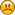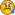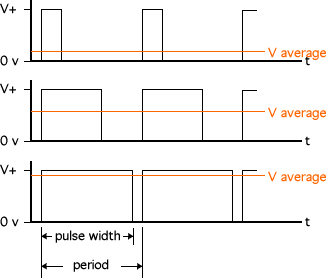###Author Topic: encoder counts to control motor speed  (Read 18370 times)

0 Members and 1 Guest are viewing this topic.

####fang-wei• Beginner
•• Posts: 6##### encoder counts to control motor speed
« on: May 10, 2007, 08:38:10 AM »
Hi!

I want to control the speed of a motor with a microcontroller (ATMEL 128)  and need to see the number of counts according to rpm, the thing is that it should not be difficult but I cannot think straight how to fix the number of counts.
DC motor vendor details:
3 pulses / revolution
360 counts / revolution
default speed: 170 rpm
I checked also that the duty cycle at this speed was 50 %
now, I should calculate the number of counts for different speeds (from 1,5 rpm till 200 rpm) ....
I will generate a PWM signal from a f cpu-clock = 8 MHz and scaled ...

Has anybody any idea?It shouldn´t be difficult !!

Greetings

fang-wei

####Admin##### Re: encoder counts to control motor speed
« Reply #1 on: May 13, 2007, 07:57:00 PM »
to start off, i recommend ignoring the fact that it is a quadrature and just use one encoder until your code works . . .

now to calculate speed . . . read the first half of this page to understand the basic concepts:
http://www.societyofrobots.com/mechanics_dynamics.shtml

you need to include the diameter of the wheel in your math . . .

wheel circumference / counts per revolution = distance traveled per encoder count

now velocity is distance divided by time . . .

velocity = (wheel circumference / counts per revolution) / time

as for generating PWM, you will have to run a PID control system and do lots of tweaking until it works . . .

####fang-wei• Beginner
•• Posts: 6##### PWM frequency
« Reply #2 on: May 14, 2007, 06:41:40 AM »
Thanks!!

I already got the encoder counts per revolution: 360 x 4 = 1440 counts / rev  if velocity is 170 rpm
it results then a 170 rev/min x 1 / 60 min/s x 1440 counts / rev = 4,079.5 counts /s    (so, frequency of 4.08 KHz )

I am not sure if I am right, but I proceed in the same way to calculate the counts / rev for different speeds (assuming the same frequency of 4.08 KHz)

The thing is that, looking at the datasheet of the microcontroller, the PWM frequency that I can generate is 60 Hz
(the formula is f pwm = fclock / 2*N*TOP  where  fclock = 8 MHz,  TOP = 65535 and N = 1 )
I think there is something wrong with this, makes any senses to use 60 Hz frequency???

I just don´t get itCan anybody help me??

Thanks!

####JonHylands##### Re: encoder counts to control motor speed
« Reply #3 on: May 14, 2007, 06:54:23 AM »
The frequency that your encoder outputs has nothing to do with the PWM frequency you run your motor at, which (in addition) has nothing to do with how fast your motor turns.

PWM frequency is one of those weird things - you need to look at the motor, the h-bridge, and figure out what works. If you're running a really high quality motor (like a Maxxon or MicroMo), you can probably run at 20 KHz. For a cheap-o plastic gearmotor, you might try 5 KHz.

The thing that sets the motor speed is the duty cycle, which is the percentage of each cycle that the PWM pin stays high.

There is no direct formula between PWM duty cycle and output speed - if there was, we wouldn't need encoders. Write a feedback loop. For simple fixed speed, you can just use a simple feedback loop that compares the computed speed (from the encoder) with the desired speed, and increases or decreases the PWM duty cycle according to how far it is off.

If you want more complex speed control, with less oscillations, look up PID on Google.

The only kind of motor where you can be guaranteed a correspondance between the driving and the actual output speed (without some kind of feedback loop) is a stepper motor.

- Jon

####Admin##### Re: encoder counts to control motor speed
« Reply #4 on: May 14, 2007, 06:56:32 AM »
Quote
I already got the encoder counts per revolution: 360 x 4 = 1440 counts / rev  if velocity is 170 rpm
it results then a 170 rev/min x 1 / 60 min/s x 1440 counts / rev = 4,079.5 counts /s    (so, frequency of 4.08 KHz )
first, '170 rpm' is not velocity of your robot, it is rotational velocity of your wheelyou MUST factor in the wheel circumference to actually calculate robot velocity

Quote
The thing is that, looking at the datasheet of the microcontroller, the PWM frequency that I can generate is 60 Hz
(the formula is f pwm = fclock / 2*N*TOP  where  fclock = 8 MHz,  TOP = 65535 and N = 1 )
I think there is something wrong with this, makes any senses to use 60 Hz frequency???
As for PWM, frequency is not that important. What is important is the wave length:Agreeing with JonHylands, there is no easy equation for PWM. The best way to implement PWM is just to do it, then tweak it until it works the way you like. You MUST have PID control - that is why you have an encoder, no?####JonHylands##### Re: encoder counts to control motor speed
« Reply #5 on: May 14, 2007, 07:23:11 AM »
I don't know where you got the number 60 Hz, btw, but the formula you posted is not correct.

On page 98 of the ATmega128 data sheet, it gives the formula (for that PWM pair) as Freq / (2 * prescalar * (1 + OCRn))

Since OCRn can be set to zero, and the prescalar can be one, that gives you a maximum frequency of 4 MHz if you're running an 8 MHz clock.

- Jon

####fang-wei• Beginner
•• Posts: 6That´s why I disccard the one you have written down...